Excel TAN Function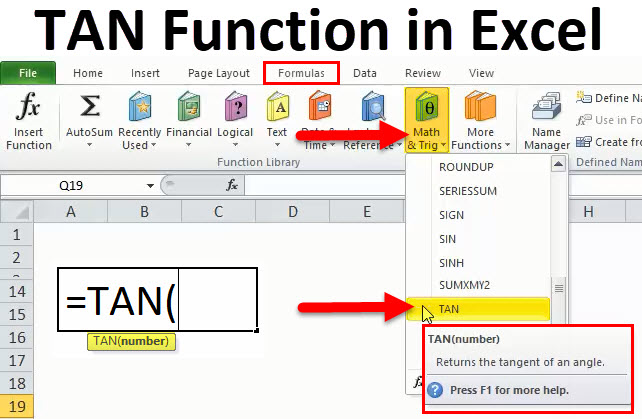• TAN in Excel
• TAN Formula in Excel
• How to Use TAN Function in Excel?

TAN in Excel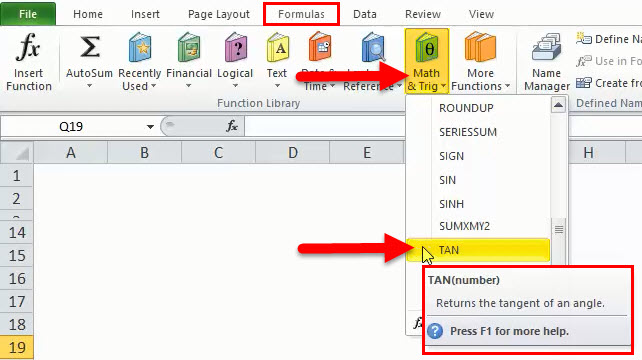The actual meaning of TAN is TANGENT. It is one of the Trigonometric functions like COS, SIN etc. TANGENT is based on right angle triangle. With the help of this Function, it is much easier to find the tangent, if we compare it with doing manually. The only thing is that we have to first find RADIANS for the given angle, then only we can easily calculate TANGENT.

All the required angle must be in Radians.

There are two ways by which we can find Radians for the given angle:

1. By using the RADIANS function inside the TAN function or first we can use RADIANS function on the degrees and then we can find the TAN from Radians. Below are calculations in Microsoft Excel in both ways: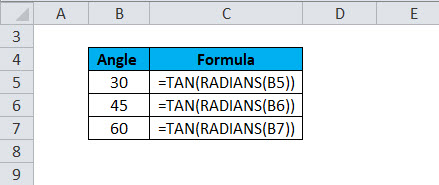OR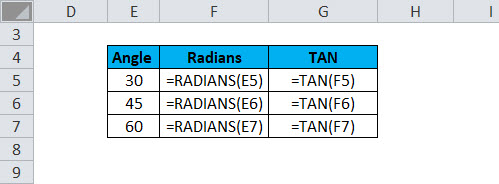1. We can also convert it in Radians by using PI function which is as: degrees * PI()/180. Below is the Excel calculation of TAN function: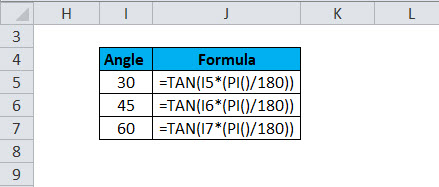The TANGENT function used in our real life as well. TANGENT is used in architectures where the calculation of length and height is required for the geometric figures. It is also used in the aeronautics, navigation system, engineering, roofing, many trigonometric applications, GPS, etc. Pilot mostly used the TANGENT function to calculate the distance from ground for landing & safety purpose.

• The TANGENT function returns the tangent of an angle and it will be always in numeric.

In Mathematics, the formula will be TAN 0 = opposite side/adjacent side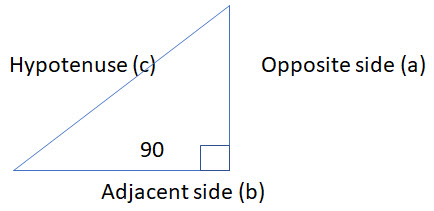So, from above pic, we can say c = a/b

TAN Formula

Below is the TAN Formula: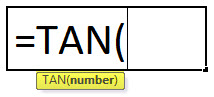Parameters or Arguments:

Number: it is the number or numeric value for which tangent needs to be calculated of an angle.

Result: TAN function always returns the numeric value after applying into a particular cell.

Type:

• Worksheet function
• VBA function

Hence, the TAN function can be used in two ways i.e. worksheet function in which the formula of TAN function needs to be entered in a particular cell of the worksheet to find the Tangent and we can also use the TAN function in VBA and we have to write the formula in Microsoft Visual Basic Editor.

How to use TAN Function in Excel?

TAN Function is very simple to use. Let us now see how to use TAN function in Excel with the help of some examples.

TAN in Excel Example #1

For calculating tangent, we have to calculate Radians so that we can have the correct data or output.

Step 1:

We have to check the raw data for which we have to calculate the tangent and verify them, below is the picture: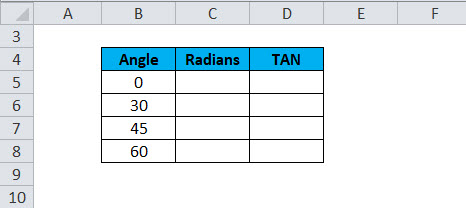Step 2:

Then click on the Formulas in the ribbon of Microsoft Excel and then we have to click on Math & Trig. Below is the picture for reference: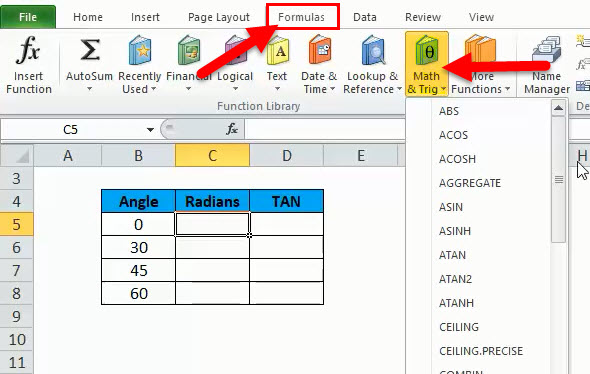Step 3:

Now, we have to apply Radians formula from the list available. So, just find RADIANS and click it as given in below picture: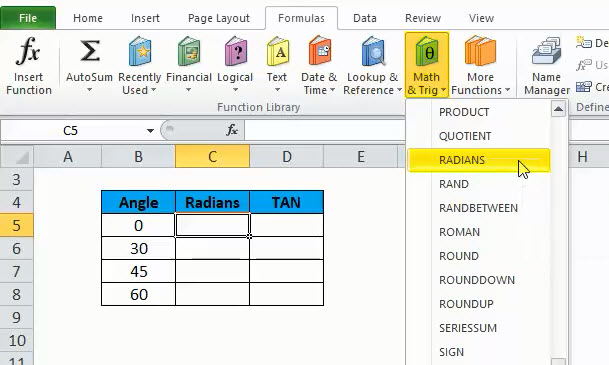Step 4:

After clicking we have like below the screen, then put the cell address or just select it from cursor for which RADIANS need to be calculated: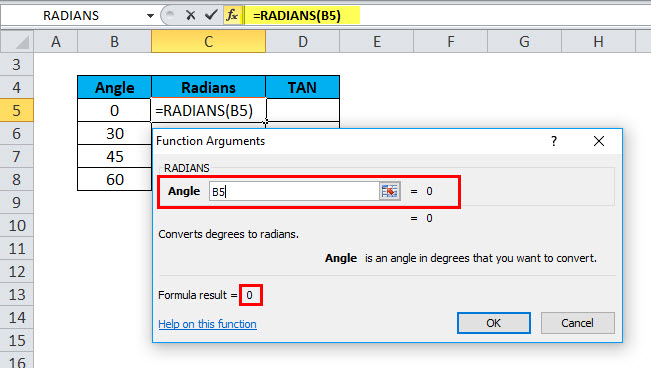So, in this case, the cell address is B5 for which RADIANS have to be calculated.

Step 5:

After selecting the cell address, we have to just click on OK or press enter, the result will be RADIANS of the given angle.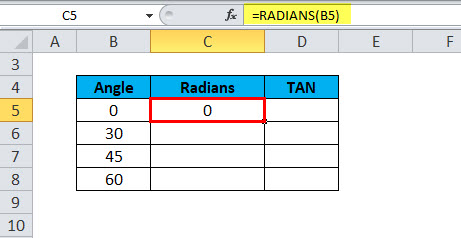The, we can drag the formula in all the required cell to find out the RADIANS. Below is the result: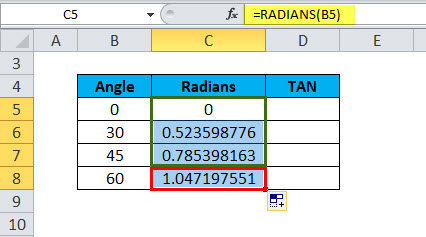Step 6:

Now, we have to calculate the TANGENT, so we can follow the steps 2 & 3 and then look for TAN and click it. Below is the picture for reference: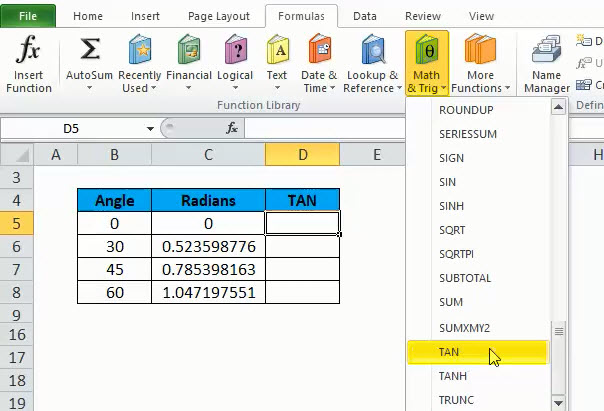Step 7:

Now we have to select the cell address for which TANGENT needs to be calculated, so as we know, we have to select the radians of given angle, then in this case we will select the cell address C5, as the argument or parameter. Below is the picture for reference: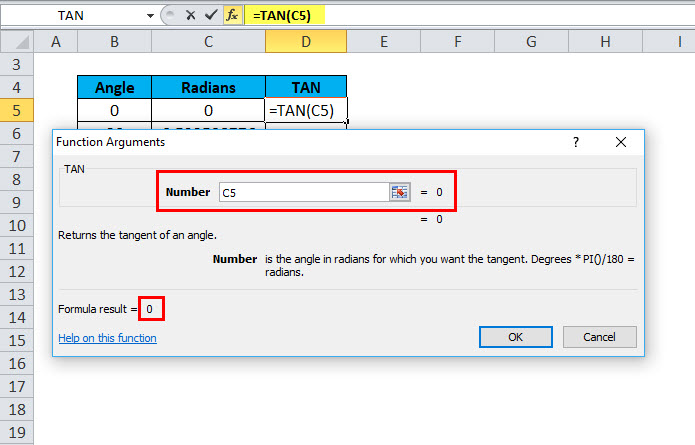Step 8:

Now, we can click ok press Enter to get the results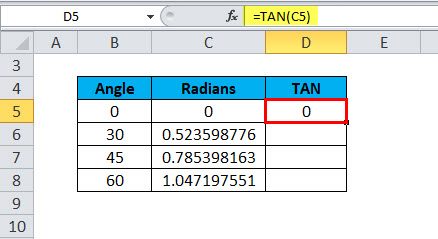and we can drag the TAN formula to another required cell as well like below: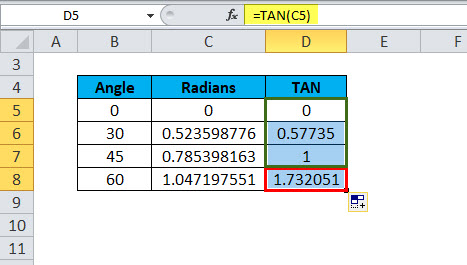So, we saw above that how to calculate TANGENT by using TAN function in Excel.

TAN in Excel Example #2

By putting formula

We can also calculate TANGENT by using formula or entering the formula of TAN in the required cell. Below is the formula:

=TAN(number)

Step 1:

Firstly, we should have Radians ready for the required angle, which we have seen above that how to calculate in step 3 to 5, which has a formula of = RADIANS(angle).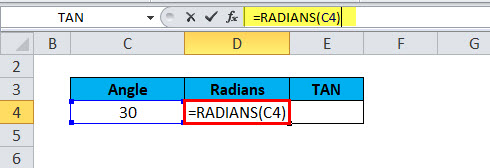The Result will be: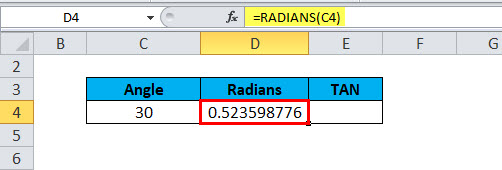Step 2:

Now, we can enter TANGENT formula in the cell address E4 which is =TAN(number). So, we have to type =TAN and then tab key. Below is the screenshot for reference: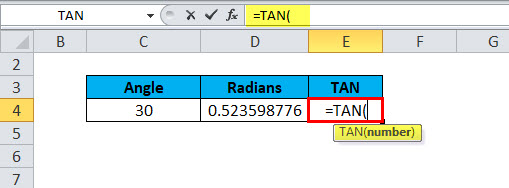Step 3:

Now, we have to select the cell address of calculated radians which is D4 in the given example, in the place of number as argument or parameter. Below is the picture for reference: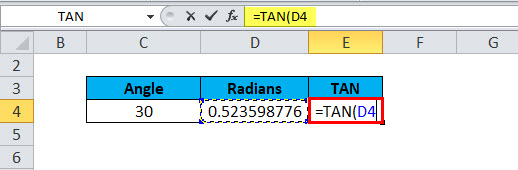Step 4:

After putting number, we have to just close the bracket to complete the formula or press enter, both have the same result. Below is the picture: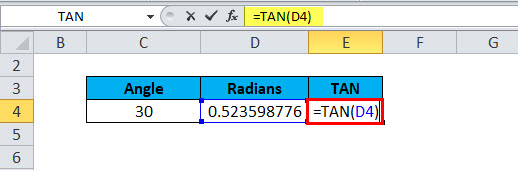Step 5:

We have the required calculated result of TANGENT after following above steps. Below is the result in the picture: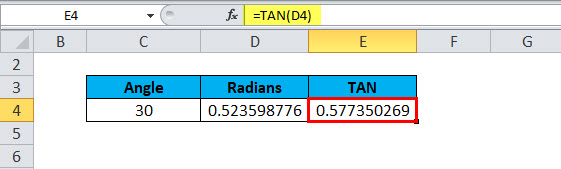TAN Function in VBA.

As we discussed above that TAN function can also be used in VBA code, so we can take one example to see that how it works and what will be the input and procedure:

Below is the VBA code to calculate Tangent:

DimLnumber As Double

LNmuber = Tan(5)

So, in the above example, the LNumber 5 contains the value of -3.380515006.

Points to Remember about TAN Function in Excel:

1. if the angle is given in degrees for which we have to calculate TAN then firstly we have to calculate RADIANS for the same by using formula =RADIAN(degree) or we can multiply the angle by PI()/180.
2. The TAN function always uses the parameter as RADIANS.
3. If the TAN function has #VALUE! An error then it means that text is used in the place of a number.
4. If the cell chooses as a blank cell in the place of a number then the result would be 1 because the tangent is 1 for the radiant 0. So, always be careful while selecting a cell.

You can download this TAN function Excel template here – TAN Function Excel Template

Recommended Articles

This has been a guide to Excel TAN function. Here we discuss the TAN Formula and how to use TAN function along with practical examples and downloadable excel template. You can also go through our other suggested articles –

1. How to Use RATE Function in Excel?
2. How to Use POWER Function in Excel?
3. How to Use SUMIF Function in Excel?
4. How to Use SUBSTITUTE Function in Excel?

The post Excel TAN Function appeared first on EDUCBA.

This post first appeared on Best Online Training & Video Courses | EduCBA, please read the originial post: here

Subscribe to Best Online Training & Video Courses | Educba

Get updates delivered right to your inbox!

×